Homework Help Question & Answers

# 2iz-1 If f(2) = 27251 2-3i a) Find and simplify f'(z) b) Find f'(1 + i)...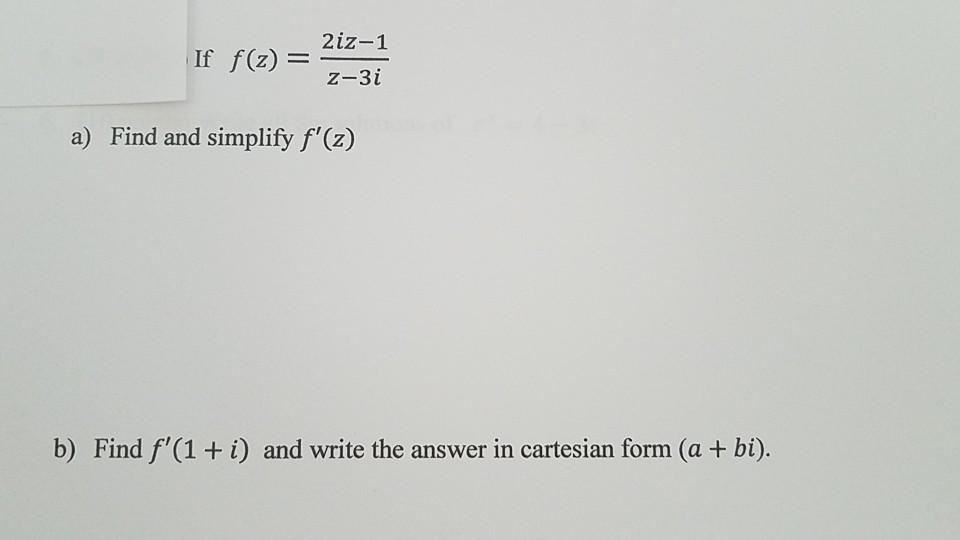2iz-1 If f(2) = 27251 2-3i a) Find and simplify f'(z) b) Find f'(1 + i) and write the answer in cartesian form (a + bi).

#### Homework Answers

Answer #1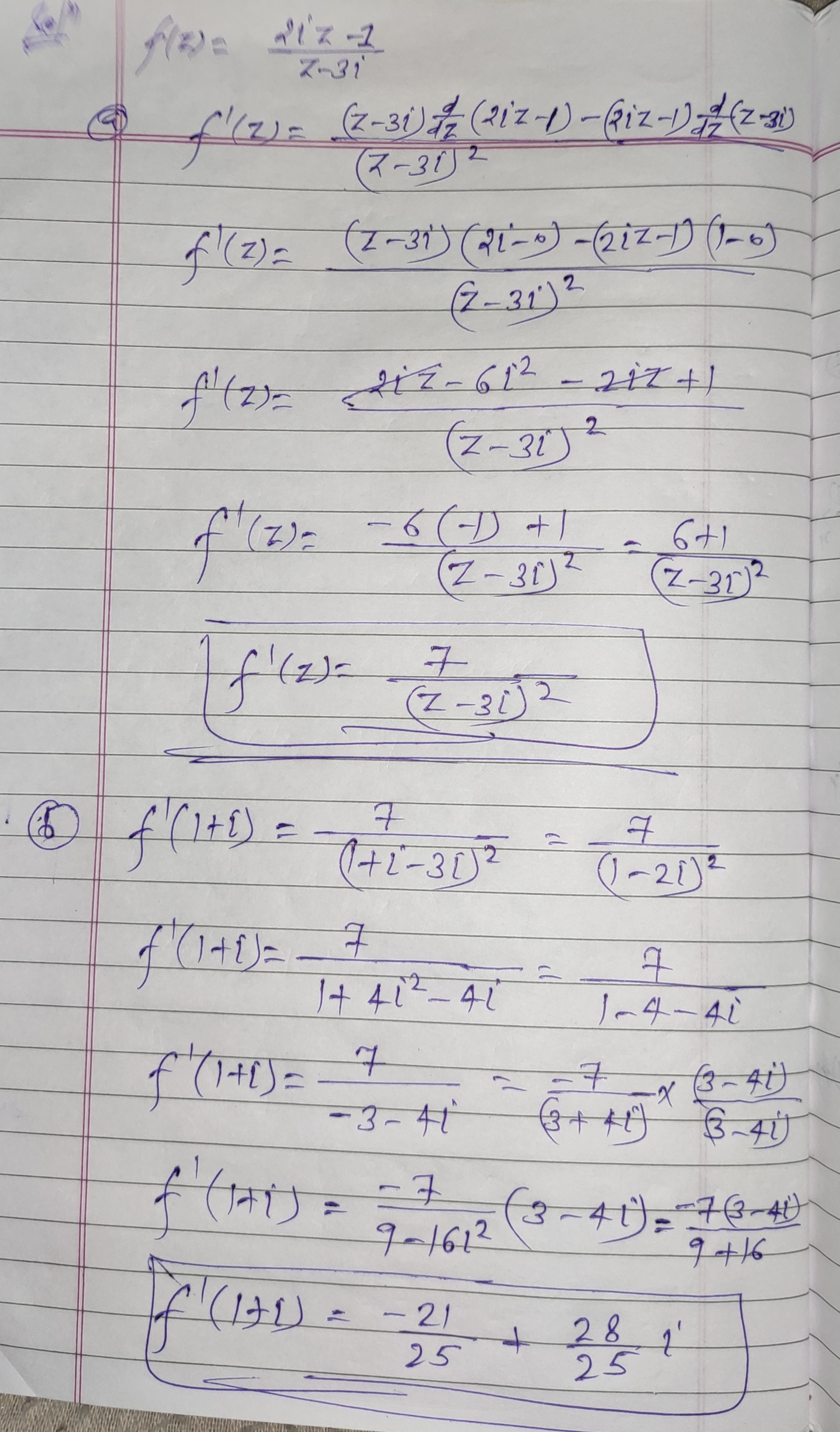Know the answer?
Your Answer:

#### Post as a guest

Your Name:

What's your source?

#### Earn Coin

Coins can be redeemed for fabulous gifts.

Not the answer you're looking for? Ask your own homework help question. Our experts will answer your question WITHIN MINUTES for Free.
Similar Homework Help Questions
• ### and z2 = 1 1 + 3i 3-i a) Given that zı = find z such...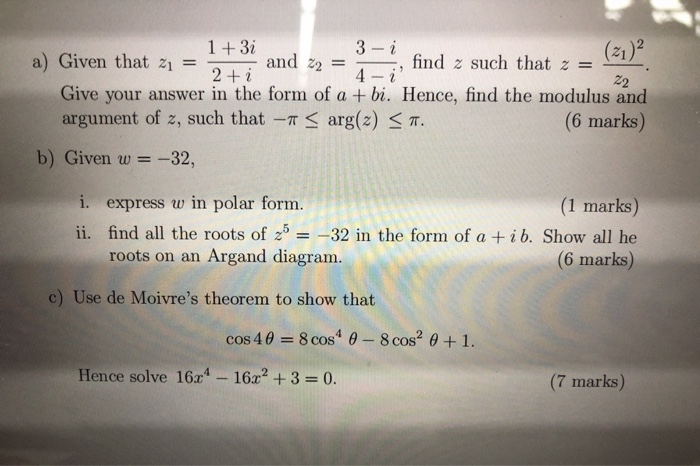and z2 = 1 1 + 3i 3-i a) Given that zı = find z such that z = 2 + i 4- ¿ 22 Give your answer in the form of a + bi. Hence, find the modulus and argument of z, such that -- < arg(2) < 7. (6 marks) b) Given w = = -32, i. express w in polar form. (1 marks) ii. find all the roots of 2b = -32 in the form of a...

• ### 4-3i 11. (8 pts) Forz 4-3i much as possible, writin work. i and w 7- i,...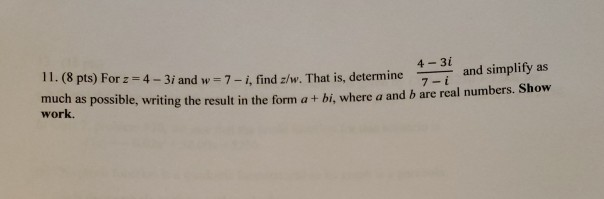4-3i 11. (8 pts) Forz 4-3i much as possible, writin work. i and w 7- i, find z/w. That is, determineand simplify as g the result in the form a + bi, where a and b are real numbers. Show

• ### Need it asap show work please Let i + 2z B(2) = 4- 2iz (a) Find...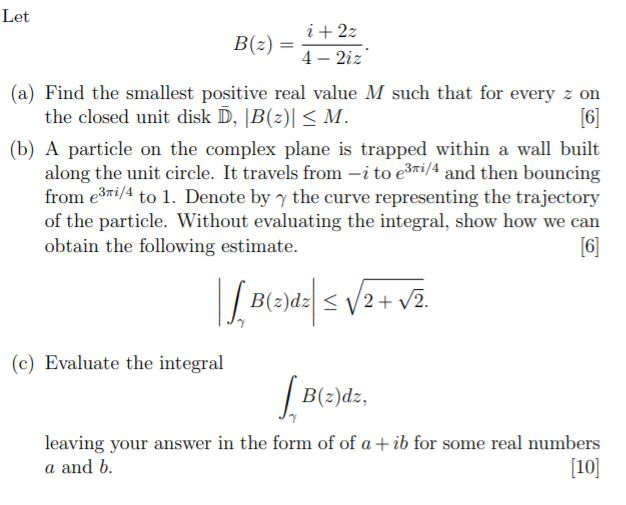Need it asap show work please Let i + 2z B(2) = 4- 2iz (a) Find the smallest positive real value M such that for every z on the closed unit disk D, B(2) < M.  (b) A particle on the complex plane is trapped within a wall built along the unit circle. It travels from -i to e3ri/4 and then bouncing from e3mi/4 to 1. Denote by the curve representing the trajectory of the particle. Without evaluating the...

• ### 6. Let B(2) i + 22 4- 2iz (a) Find the smallest positive real value M...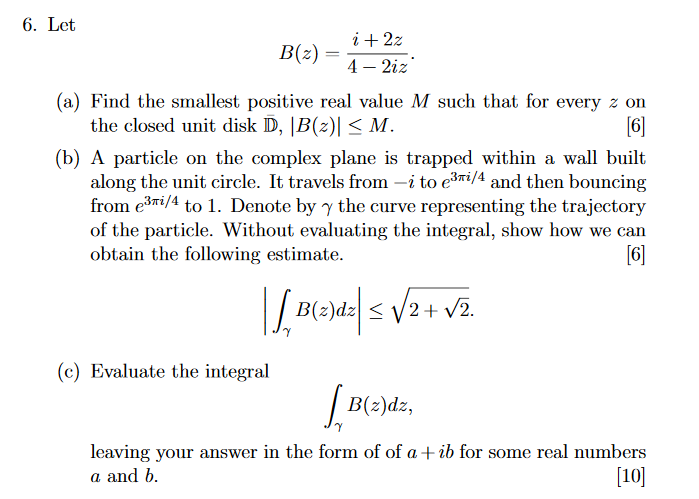6. Let B(2) i + 22 4- 2iz (a) Find the smallest positive real value M such that for every z on the closed unit disk D, B(2) <M.  (b) A particle on the complex plane is trapped within a wall built along the unit circle. It travels from -i to e3ri/4 and then bouncing from e3mi/4 to 1. Denote by y the curve representing the trajectory of the particle. Without evaluating the integral, show how we can obtain...

• ### 6. Let B(2) = i + 2z 4 - 2iz (a) Find the smallest positive real...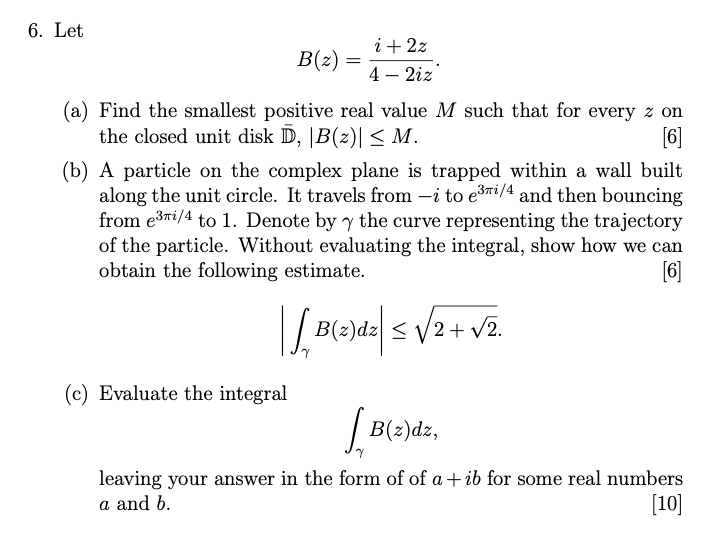6. Let B(2) = i + 2z 4 - 2iz (a) Find the smallest positive real value M such that for every z on the closed unit disk D, |B() < M.  (b) A particle on the complex plane is trapped within a wall built along the unit circle. It travels from –i to e3ri/4 and then bouncing from e3vi/4 to 1. Denote by y the curve representing the trajectory of the particle. Without evaluating the integral, show how...

• ### Question 1) Find I = z +2 3z - 2 + 3i 22 + (2i -...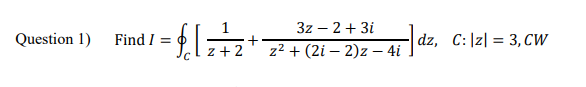Question 1) Find I = z +2 3z - 2 + 3i 22 + (2i - 2)2 - 4i ] dz, C:\z| = 3, CW a. 4πί b. 8πί C. 2πί d. -2π(3 +i) e. 0.0 f. ο g. -4πί h. 6π

• ### Let B(2) = i + 22 4 – 2iz. (a) Find the smallest positive real value...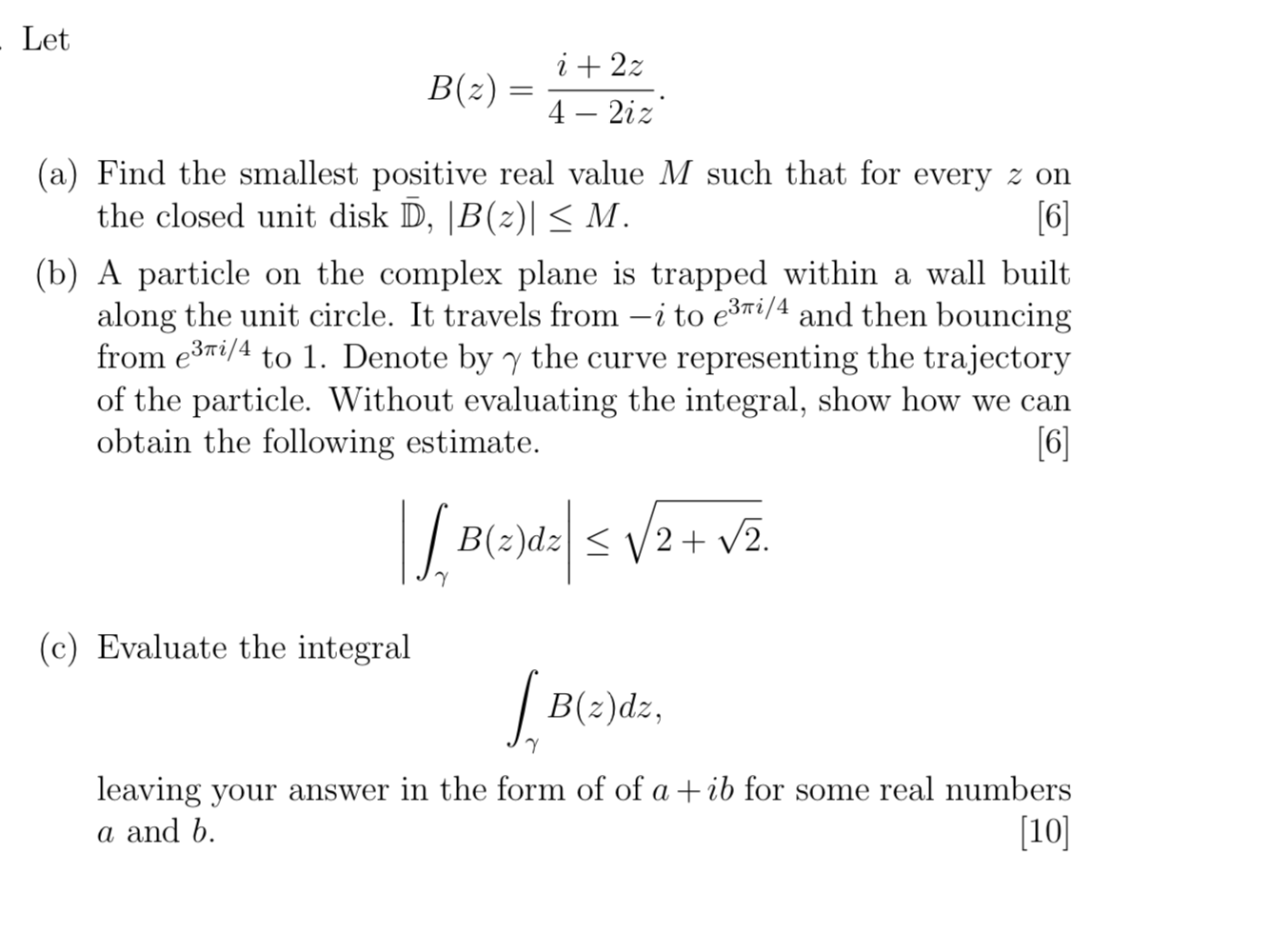Let B(2) = i + 22 4 – 2iz. (a) Find the smallest positive real value M such that for every z on the closed unit disk D, 5B() < M.  (b) A particle on the complex plane is trapped within a wall built along the unit circle. It travels from –i to e3ti/4 and then bouncing from e3ti/4 to 1. Denote by y the curve representing the trajectory of the particle. Without evaluating the integral, show how we...

• ### Let B(2) = i + 22 4 – 2iz. (a) Find the smallest positive real value...Let B(2) = i + 22 4 – 2iz. (a) Find the smallest positive real value M such that for every z on the closed unit disk D, 5B() < M.  (b) A particle on the complex plane is trapped within a wall built along the unit circle. It travels from –i to e3ti/4 and then bouncing from e3ti/4 to 1. Denote by y the curve representing the trajectory of the particle. Without evaluating the integral, show how we...

• ### Find the complex numbers w and z which solve the system of equations (-1+i)w + (-2-3i)z...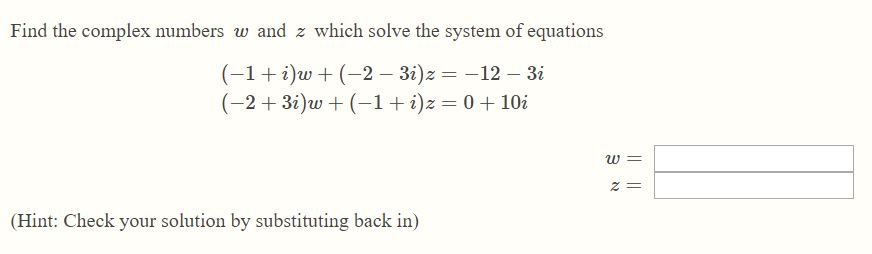Find the complex numbers w and z which solve the system of equations (-1+i)w + (-2-3i)z = -12 - 3i (-2+3i)w +(-1+i)z = 0 +10i (Hint: Check your solution by substituting back in)

• ### divide: 2+3i-3i^3/1-i 1-2i+i^3/1+i simplify i^16/i^3

divide: 2+3i-3i^3/1-i1-2i+i^3/1+isimplify i^16/i^3

Free Homework App

Scan Your Homework
to Get Instant Free Answers
Need Online Homework Help?

Get Answers For Free
Most questions answered within 3 hours.